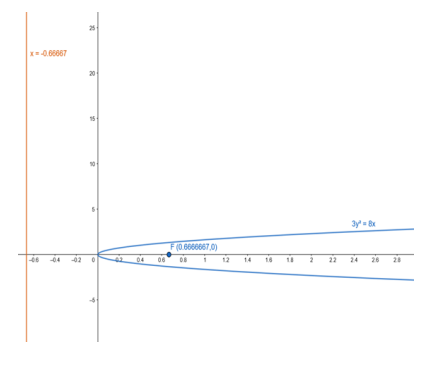Deepak Scored 45->99%ile with Bounce Back Crack Course. You can do it too!

# Find the coordinates of the focus and the vertex, the equations of the

Question:

Find the coordinates of the focus and the vertex, the equations of the directrix and the axis, and length of the latus rectum of the parabola:

$3 y^{2}=8 x$

Solution:

Given equation :

$3 y^{2}=8 x$

$y^{2}=\frac{8}{3} x$

Comparing the given equation with parabola having equation,

$y^{2}=4 a x$

$4 a=\frac{8}{3}$

- $a=\frac{2}{3}$

Focus: $F(a, 0)=F\left(\frac{2}{3}, 0\right)$

Vertex : $A(0,0)=A(0,0)$

Equation of the directrix : $x+a=0$

$x+\frac{2}{3}=0$

$\mathrm{x}=-\frac{2}{3}$

Lenth of latusrectum : $4 a=\frac{8}{3}$